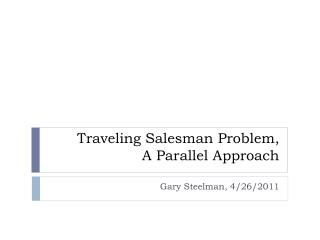# Traveling Salesman Problem, A Parallel Approach - PowerPoint PPT PresentationDownload PresentationTraveling Salesman Problem, A Parallel Approach

Traveling Salesman Problem, A Parallel ApproachDownload Presentation## Traveling Salesman Problem, A Parallel Approach

- - - - - - - - - - - - - - - - - - - - - - - - - - - E N D - - - - - - - - - - - - - - - - - - - - - - - - - - -
##### Presentation Transcript

1. Traveling Salesman Problem,A Parallel Approach Gary Steelman, 4/26/2011

2. Definitions and Setup

3. Problem Definition • The Traveling Salesman Problem (TSP) says that given an arbitrary number of cities… • Start in a random city • Visit each city exactly once • Return to initial city after completion of tour • Minimize cost of tour

4. So Really… • Create a connected, weighted graph G • Vertices (nodes) in G represent cities • Edges represent paths between cities • Edge weights represent cost of moving from one city to another • Edges may be directed or undirected • Usually represented with an adjacency matrix • Create a Hamiltonian cycle for G • Initial city is random • Minimize total cost of edges traversed • Resulting cycle is the same regardless of initial city • NP-Complete non-linear optimization problem

5. Example Graph Undirected, weighted graph with 5 cities labeled A through E.

6. Algorithms for Solving Optimization Problems

7. Optimization Algorithms • Genetic Algorithms (GA) • Candidate solutions are modeled using genetics and improved using recombination and mutation • Simulated Annealing (SA) • Local search each iteration with acceptance of adjacent solutions based on temperature • Particle Swarm Optimization (PSO) • Candidate solutions are relocated in search space, movement governed by simple formulae • Ant Colony Systems (ACS) • As a candidate solution is created each iteration, its edges’ pheromone levels are increased

8. Parallel GA for TSP (Version 1) • Each thread • Generate N random solutions • Loop X number of generations • Calculate fitness of current population • Select two best solutions mate them using recombination and mutation • Generate random solutions for the new population • Reduce best results to master thread • Essentially the exact same algorithm as sequential, but executed within each thread

9. Parallel GA for TSP (Version 2) • Master thread • Generate N random solutions • Loop X number of generations • Send a solution to a slave thread for fitness evaluation • Receive evaluated fitness from each slave • Perform recombination and mutation for best solutions • Generate random solutions for new population • Slave thread • Loop until termination signal from master • Wait for solution from master thread • Evaluate fitness of solution • Send fitness to master thread

10. Parallel GA for TSP Analysis Version 1 (N-Sequential) Version 2 (Master-Slave) • Speedup • O(P), linear • Efficiency • O(1) • Speedup • O(P), linear • Efficiency

11. Parallel SA for TSP (Version 1) • Each thread • Generate a random solution • Loop X number of iterations • Generate neighbor route by exchanging a pair of cities • Accept/Decline neighbor route • Reduce best results to master thread • Essentially the exact same algorithm as sequential, but executed within each thread

12. Parallel SA for TSP (Version 2) • Master thread • Generate P random solutions, send to slave threads as initial solutions • Then perform slave thread logic • Slave thread • Loop X number of times • Receive initial solution from master or other slaves • Perform sequential SA on solution • Exchange solution with other slaves, all slaves keep best partial solution • Send best partial result found to master

13. Parallel SA for TSP Analysis Version 1 (N-Sequential) Version 2 (Master-Slave) • Speedup • O(P), linear • Efficiency • O(1) • Speedup • O(P), linear • Superlinear for P ≥ 9 • Efficiency • P/(P-1) = O(1) for large P

14. Parallel ACS for TSP • Each thread • Loop X iterations • Each worker starts at a random node • Loop Y steps • Each worker applies state transition rule to build local solutions, choice to move to adjacent city influenced by pheromone on edges • Each worker applies local pheromone update rule • Apply 3-opt heuristic to bring each worker to local minimum • Apply global pheromone update rule • After X iterations, tour with most pheromone is optimal • Global Update: • Only the best ant is allowed to deposit pheromone into global solution set

15. Parallel ACS for TSP Analysis • Speedup • Not reported • Efficiency • Not reported • Completes faster (seconds) with less relative error (tour length) versus genetic algorithms, evolutionary algorithms, and simulated annealing

16. Conclusions and Summary • Unless a sequential implementation is executed P times in parallel, efficiency decreases due to communication overhead • Linear speedup is possible • Parallel ACS with a “good” initial guess is extremely fast

17. Questions? End of Presentation

18. Sources • Yang, Fan. “Solving Traveling Salesman Problem Using Parallel Genetic Algorithm and Simulated Annealing.” Massachusetts Institute of Technology . 18 May 2010. Web. 15 Mar 2010. <http://beowulf.lcs.mit.edu/18.337/projects/reports/Fan_Yang_Final_Project_Report_Fixed_Figure_References.pdf> • Borovska, Plamenka. “Solving the Travelling Salesman Problem in Parallel by Genetic Algorithm on Multicomputer Cluster.” International Conference on Computer Systems and Technologies. 2006. Web. 15 Mar 2010. <http://ecet.ecs.ru.acad.bg/cst06/Docs/cp/SII/II.11.pdf> • D. Janaki Ram, T.H. Sreenivas, and K. GanapathySubramaniam. “Parallel Simulated Annealing Algorithms.” Department of Computer Science and Engineering, Indian Institute of Technology. 1996. Web. 15 Mar 2010. <http://citeseerx.ist.psu.edu/viewdoc/download?doi=10.1.1.100.6565&rep=rep1&type=pdf> • Marco Dorigo, Luca Maria Gambardella. “Ant Colony System: A Cooperative Learning Approach to the Traveling Salesman Problem.” University Libre de Bruxelles, Belgium. 1997. Web. 15 Mar 2010. <http://www.idsia.ch/~luca/acs-ec97.pdf> • Shu-Chuan Chu, John F. Roddick, Jeng-Shyang Pan, Che-Jen Su. “Parallel Ant Colony Systems.” School of Informatics and Engineering, Flinders University of South Australia. 2003. Web. 15 Mar 2010. <http://books.google.com/books?id=41dm6jorJkwC&lpg=PA279&ots=EmjfAU75oj&lr&pg=PA279#v=onepage&q&f=false> • http://math.berkeley.edu/~sethian/2006/Applications/TravelingSalesmen/traveling_figure.tran.gif